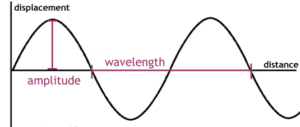Courses

# Displacement Relationship in a Progressive Wave Class 11 Notes | EduRev

## JEE : Displacement Relationship in a Progressive Wave Class 11 Notes | EduRev

The document Displacement Relationship in a Progressive Wave Class 11 Notes | EduRev is a part of the JEE Course Physics For JEE.
All you need of JEE at this link: JEE

The waves of water or waves on a string travel in a particular direction. But, have you ever imagined how is this direction decided? What is the influence of this movement? In order to understand this concept in-depth, it is important to learn about progressive wave and its displacement aspect.

Progressive Wave

A progressive wave is a term given to a wave that travels from a specific point A in the medium to another point B. In simple terms, a wave that continuously travels in a medium in the same direction minus the changes is known as a traveling wave or progressive wave. Furthermore, a progressive wave is of two types; namely, transverse wave and longitudinal wave.• Transverse Waves: These are waves in which the particle displacement in the medium is measured perpendicular to the wave direction or direction of wave travel.
• Longitudinal Waves: With Longitudinal Waves, the particle displacement remains in the same direction to the wave travel.

Plane Progressive Harmonic Wave
During the transmission of a wave via a medium, the particles present in the medium tend to vibrate harmonically about their mean positions; in this case, the wave is tagged as a plane progressive harmonic wave.

Simple Harmonic Progressive Wave

This waveform continuously travels in a specific direction without any transition in form. Furthermore, the particles of the medium tend to move harmonically about their mean position carrying the same amplitude and period.

Characteristics of SHM Wave

• Note that, all particles or components of medium exhibit SHM while the wave passes through a medium.
• The same amplitude is maintained by all particles as they vibrate.
• Energy is conducted through the medium.
• The total number of particles vibrate with a similar frequency.

Plane Progressive Harmonic Wave: Displacement Relation

Considering a plane progressive harmonic wave, the displacement of a sinusoidal wave traveling in the x-direction (positive) is mentioned below:
y = a sin(kx – ωt + φ)

Here, ‘a’ denotes the amplitude of the wave, angular wave number is denoted by ‘k’, whereas ‘ω’ is the angular frequency. The phase is mentioned as (kx – ωt + φ), and φ signifies the phase angle or phase constant. Furthermore, the sine function, as well as the time-dependent phase (wave), resemble the oscillation of a string component, whereas the amplitude of wave defines the extremes of the component’s displacement. It is important to remember that, the constant ‘ϕ’ is termed as initial phase angle.

Wavelength of Progressive Wave

The wavelength ‘λ’ for a progressive wave is basically the distance measured between two succeeding points of the same phase at a particular time. Considering a stationary wave, this is twice the distance measured between two successive nodes or antinodes. The propagation constant is defined as ‘k’. The SI unit is calculated in radian per meter or rad m-1.

k=2π/λ

Frequency and Period of Progressive Wave

The time period ‘T’ of wave oscillation is the duration taken by any component of the medium takes to travel over one complete oscillation. This is related to ‘ω’ or angular frequency through the following relation:
ω = 2π/T
In this, wave frequency ‘v’ is mentioned as 1/T and is also related to angular frequency as:

v = ω/2π

It can also be defined as the number of oscillations/unit time prepared in a string element while the wave passes through it. This is usually calculated in Hertz.

Offer running on EduRev: Apply code STAYHOME200 to get INR 200 off on our premium plan EduRev Infinity!

## Physics For JEE

188 videos|517 docs|265 tests

,

,

,

,

,

,

,

,

,

,

,

,

,

,

,

,

,

,

,

,

,

;